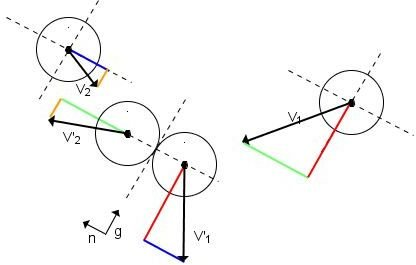# Momentum – Theory of Conservation of Momentum – Elastic & Inelastic Collisions

## What is Momentum?

Momentum is a physical quantity related to the motion and the mass of a specific object. The formula for momentum is:

p = m x v

where p is the momentum [kg·m/s], m the mass [kg] of the object and v the velocity [m/s] of the object. All units are in the S.I. Momentum and velocity are both vector quantities, meaning that they also have directional dimensions.

The formula is valid as far as classical physics is concerned. A more universal expression for momentum is given by Newton's second law, where the rate of change of the momentum dp/dt of an object is proportional to the resultant force F acting on the object and is in the direction of that force (vectors):

F = dp/dt

So Newton's second law is a fine expression for relating the physical quantities of force and momentum.

But what is the meaning of momentum? In order to understand the physical meaning of momentum, we will have to use an example. A car with a mass of 1,000 kg hits a wall at a speed of 100 m/s causing certain damage. The same damage will be caused by a big truck with a mass of 10,000 kg speeding at 10m/s only. This is because in both cases the momentum of the two vehicles is the same.

## Laws of Conservation – The Principle of Conservation of Momentum

Some of the most fundamental laws of the universe have to do with the conservation principles of energy, momentum, and angular momentum. More specifically, the law of conservation of momentum indicates that if no external force acts on a closed system of objects the momentum of the closed system remains constant.

Although the momentum of each individual object within the closed system may change, the overall momentum of the system will stay the same. If an external force is applied to the system, then the overall momentum will change. This fact is also described by Newton's first law or principle of inertia, where an object tends to continue moving at a constant speed, unless some external forces causes it to change its speed or direction.

The conservation of energy principle applies in the case of elastic and inelastic collisions as described below.

Image: In the case of an elastic collision between two objects of equal masses, there is simply an exchange in velocities. This is a result of the conservation of momentum principle and can be demonstrated by Newton's cradle.## Types of Collisions

The phenomenon in which momentum and/or kinetic energy is transferred from one object to another is called collision. The simplest system deals with two objects colliding. There are two main types of collision: elastic and inelastic.

In the case of elastic collision, both the kinetic energy K and momentum p are conserved. This means that the sum of the momenta before the collision will be equal to the sum of momenta after the collision. The same applies for the kinetic energy. At this point it is helpful to remember the related formulas:

p = m x v

K = (1/2) x m x v^2

It is obvious that both of these quantities are related to the motion of the object and both depend on its mass m and velocity v. However, their relationship is different. Although both quantities have a linear dependence on the mass, they differ in the velocity dependence. The kinetic energy is exponentially related to the velocity. This means that if the velocity of an object is doubled, its momentum will be doubled as well, whereas its kinetic energy will increase by a factor of four. The action of billiard balls is a good example of elastic collision.

In the case of inelastic collision, only the momentum is conserved. What is happening to the kinetic energy is that an amount of this energy is lost in a variety of ways, such as heat (through friction) and deformation. If we could add up the remaining kinetic energy and the energy lost or transferred to the environment, it would equal the original amount of kinetic energy.Most examples in everyday life are inelastic collisions, including car accidents, a bullet hitting a target, etc.

For more information on how to solve elastic and inelastic collision problems, check out the articles, How to Solve Elastic Collision Problems and How to Solve Inelastic and Plastic Collision Problems.

## Sources:

• Momentum Principle, Dept of Earth Sciences, Uppsala Universitet
• More on the Momentum Principle, Dept of Physics, Drexel University of Arts and Science
• Types of Collisions, by D. Newman, Dept of Phycics, University of Alaska

Image Credits:

• Explanation of Conservation of Momentum Through Newton's Cradle (en.wikipedia.org)
• Elastic Collision of Two Spherical Bodies of the Same Mass (commons.wikimedia.org)